Search IntMath
Close

450+ Math Lessons written by Math Professors and Teachers

5 Million+ Students Helped Each Year

1200+ Articles Written by Math Educators and Enthusiasts

Simplifying and Teaching Math for Over 23 Years

# IntMath Newsletter: trig differentiation applet, art, music

By Murray Bourne, 24 Jan 2017

24 Jan 2017

1. Differentiation applet - trigonometric functions
2. Math art
3. Resource: SimplexNumerica
4. Math movie: Music And Measure Theory
5. Math puzzles
6. Final thought: Challenges

Happy New Year and Gong Xi Fa Cai!

Today's Newsletter has a cultural (art & music) focus.

## 1. Differentiation interactive applet - trigonometric functions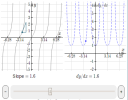In this applet you can explore the shapes of the six trigonometric functions (sin, cos, tan, csc, sec and cot) and their derivatives. See: Differentiation Interactive Applet - trigonometric functions

You can see some background on this interactive in these three earlier articles:

Explore the slope of the sin curve

## 2. Math art

I spotted this great piece of 3D art based on mathematical principles at a recent exhibition at the Art Science Museum in Singapore recently. It's like warped sine curves projecting out from a surface.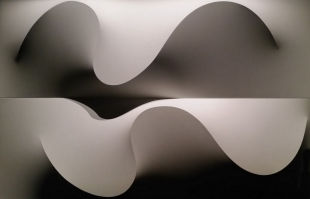For me, it's a soothing image which promotes reflection.

## 3. Resource: SimplexNumerica

Here's a useful zero-cost offering: SimplexNumerica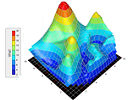SimplexNumerica produces high quality 3D math graphics, including surface plots, data analysis and visualization; and 2D contour, Gantt, finance and vector charts. See: SimplexNumerica

Here are some sample screen shots, showing a contour plot and a polar vector plot.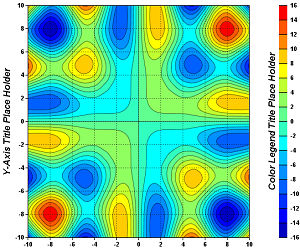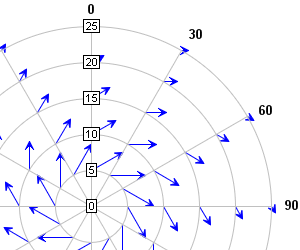[Images: source]

SimplexNumerica is available in 32-bit and 64-bit Windows versions (but no Mac or Linux versions, unfortunately).

## 4. Math movie: Music And Measure Theory

There's a very clever series of videos that relate math concepts in animations over at 3blue1brown. I'm going to feature some of them here and in future Newsletters.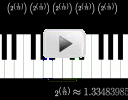Music and Measure Theory ties in Pythagorean ratios in music (why do some chords sound better than others?) with covering a dense set in the reals with open intervals whose lengths sum to an arbitrarily small constant.

It sounds scary, but it's very well illustrated and for the most part, easy to follow. See:

Music And Measure Theory

## 5. Math puzzles

The puzzle in the last IntMath Newsletter asked about writing the digits of 2017. There were no submissions, but I'll leave it open. This task could be a good class exercise for practicing mental number operations.

### New math puzzle: Geometric progression

Find the least number of terms of the following geometric progression such that 2 − N < 0.0001.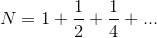You can leave your responses here.

## 6. Final thought: challenges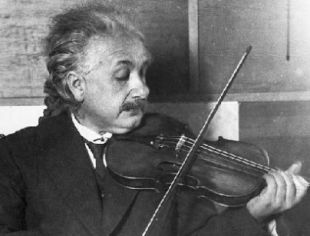Image source

It often seems that happiness comes from enjoyable but challenging pleasures, and involves few possessions. This is what Einstein had to say about it:

A table, a chair, a bowl of fruit and a violin; what else does a man need to be happy? [Albert Einstein]

Until next time, enjoy whatever you learn.

See the 9 Comments below.

### 9 Comments on “IntMath Newsletter: trig differentiation applet, art, music”

0.0001>2-N
N>1.9999
a=1, r=½
N =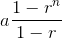> 1.9999
After substituting each value;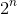> 20000
Taking the log of both sides;
nlog2 > log20000
n >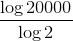n > 14.2877
Least number of terms is greater than 14.2877, say 15

2. Bruce Tivendale says:

Using an Excel spreadsheet, 15 terms.

3. Aksel Soee says:

2 -(1-.5^15)/.5 < 0.0001
The answer is 15

4. Malcolm says:

Hello Murray and thanks for the mails. I puzzle at some of the challenges but progress with few. But it is good exercise for the gray matter...
I went as far as I could to solve the geometric progression puzzle, approaching it from looking for n by using the formula
Sn=a1 . 1-rn but could not get it to work out
-----
1-r

Also tried 1.99991= a1.r to power n-1 (i think...) but seemed to progress only to 0.999999 not 1.99991 which is where I needed to get to (I think...).
I will enjoy seeing the answer and method next month....

Malcolm

5. Ed Harris ( Mr. Ed ) says:

SimplexNumerica is Da Bomb !!! Thank you so much, I'm planning to have loads of fun this weekend plotting vector functions. So much Mathematics, so little time !!!

6. Tomas Garza says:

Assuming that the general term of the series is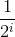I calculate the first 14
terms of the sum, starting with i = 0, and find that the differences from 2 are

{{1, 1.}, {2, 0.5}, {3, 0.25}, {4, 0.125}, {5, 0.0625}, {6 ,0.03125}, {7, 0.015625}, {8, 0.0078125}, {9, 0.00390625}, {10, 0.0019 5313}, {11, 0.000976563}, {12, 0.000488281}, {13, 0.000244141}, {14 ,0.00012207}, {15, 0.0000610352}

Each of the pairs above contains the number of terms (the sum starting from 0), and the difference. The number of terms required is 15.

7. don miller says:

ONE 5 LINE FOR/NEXT WAS USED
FOR N=0 TO 15
X =1/(2^N)
S =S+X
PRINT 2-S,,N
NEXT

8. Eamon Day says:

Puzzle Solution.

Taking N to mean the sum of the series to n terms, in this case the first term = 1 and common factor = 0.5. Then by standard formula N=(1-(0.5^n))/(1-0.5)

If 2-N<0.0001, then 1.99991.9999

Start by letting N=1.9999. Then 1.9999=(1-(0.5^n))/0.5
therefore 1-(0.5^n)=0.99995
hence 0.5^n= 0.00005
Taking logarithms of each side:
n ln(0.5)=ln(0.00005)
n=ln(0.00005)/ln(0.5) = -9.9035/-0.69315 = 14.28

n must be an integer and N>1.9999, therefore n=15

Check: when n=15, N=(1-(0.5^15))/0.5 = 1.99994

If we let n=14 then N is 1.99988 which is too small.

Therefore the least number of terms is 15.

9. Gerard Grufferty says:

IntMath puzzle for January 2017

2 - N 1·9999

Use the geometric series formula sum to n terms
a(1 - r^n) / (1 - r^n) = 1(1 - 0·5^n)/(1 - 0·5) > 1·9999

1 - 0·5^n > 0·99995

0·5^n > 0·00005

2^n < 20000

n log 2 < log 20000

n < (log 20000)/ log 2

n < 14·28771238

Therefore n = 14
14 terms will suffice.

### Comment Preview

HTML: You can use simple tags like <b>, <a href="...">, etc.

To enter math, you can can either:

1. Use simple calculator-like input in the following format (surround your math in backticks, or qq on tablet or phone):
a^2 = sqrt(b^2 + c^2)
(See more on ASCIIMath syntax); or
2. Use simple LaTeX in the following format. Surround your math with $$ and $$.
$$\int g dx = \sqrt{\frac{a}{b}}$$
(This is standard simple LaTeX.)

NOTE: You can mix both types of math entry in your comment.